# 7 Time and Distance Important Formulas and Shortcut Tricks

By | March 5, 2022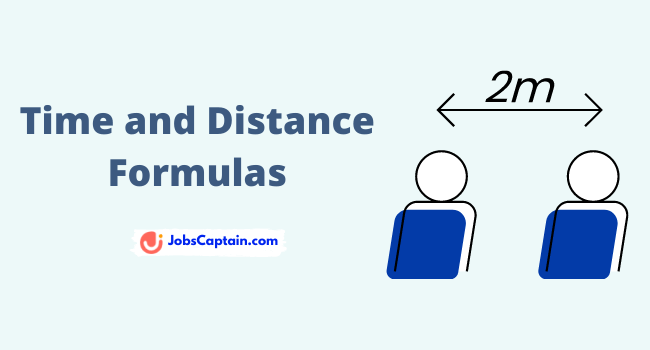Time and Work is the topic of Quantitative Aptitude. In each and every Sarkari exam, there will always be 3-4 questions asked on this topic.

Read the Time and Distance important maths formulas and equations to solve the questions easily and score more marks in your competitive exams.

In this post, you will get time and work formulas, shortcut tricks and tips to solve speed questions.

 Topic Name Time and Distance Formulas Total Formulas 7+ Language English

## Time and Distance Formulas

We have uploaded all Time and Distance Formulas from all chapters.

Let’s read these 7 Time and Distance Formulas:

1. Formula 1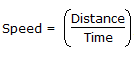2. Formula 2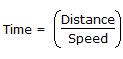3. Formula 3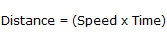4. km/hr to m/sec conversion: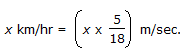5. m/sec to km/hr conversion: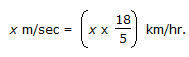6. If the ratio of the speeds of A and B is a : b, then the ratio of the times taken by then to cover the same distance is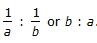7. Suppose a man covers a certain distance at x km/hr and an equal distance at y km/hr. Then, the average speed during the whole journey is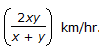Thank you for reading Time and Distance Important Formulas. We hope these formulas will help you to solve Time and Distance related questions easily and in a speedy manner.

Maths Formulas:

Furthermore, if you have any questions regarding this topic then do comment below.

Category: Maths PDF